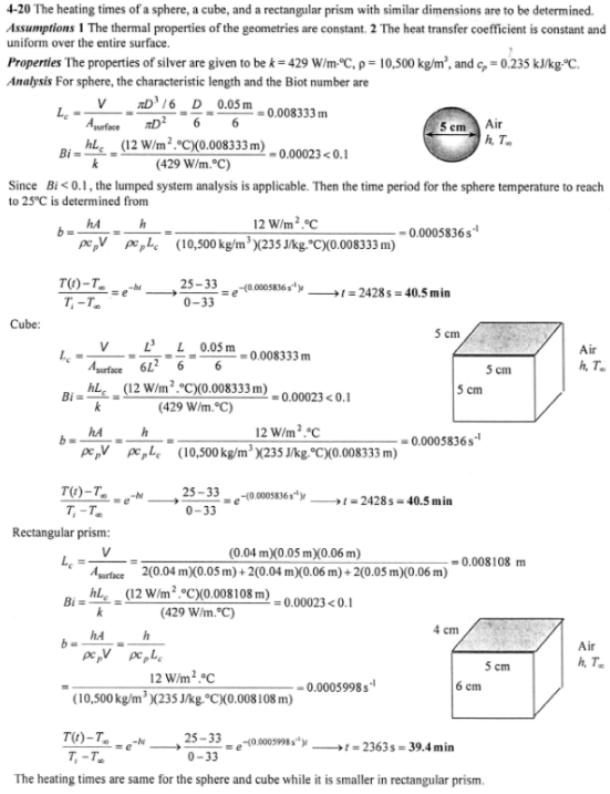heat and mass transfer problems engineering equations heat and mass transfer school homework engineering heat and mass transfer formulas heat and mass transfer solutions to heat and mass transfer problems full solution engineering problem solution heat and mass transfer math problems engineering equations heat and mass transfer school homework engineering solutions to heat and mass transfer formulas heat problems mass problem solutions to transfer problems full solution heat and mass transfer
heat and mass transfer problems engineering equations heat and mass transfer school homework engineering heat and mass transfer formulas heat and mass transfer solutions to heat and mass transfer problems full solution engineering problem solution heat and mass transfer math problems engineering equations heat and mass transfer school homework engineering solutions to heat and mass transfer formulas heat problems mass problem solutions to transfer problems full solution heat and mass transfer
Highalphabet Home Page heat and mass transfer problem solutions Heat and Mass Transfer Page
Consider a sphere of diameter 5 cm, a cube of side length 5 cm, and a rectangular prism of dimension 4 cm x 5 cm x 6 cm, all initially at 0 C and all made of silver (k=429 W/mK, rho=10500 kg/m^3, c_p=0.235 kJ/kgK). Now all three of these geometries are exposed to ambient air at 33 C on all of their surfaces with a heat transfer coefficient of 12 W/m^2 K. Determine how long it will take for the temperature of each geometry to rise to 25 C.Consider a sphere of diameter 5 cm, a cube of side length 5 cm, and a rectangular prism of dimension 4 cm x 5 cm x 6 cm, all initially at 0 C and all made of silver (k=429 W/mK, rho=10500 kg/m^3, c_p=0.235 kJ/kgK). Now all three of these geometries are exposed to ambient air at 33 C on all of their surfaces with a heat transfer coefficient of 12 W/m^2 K. Determine how long it will take for the temperature of each geometry to rise to 25 C.